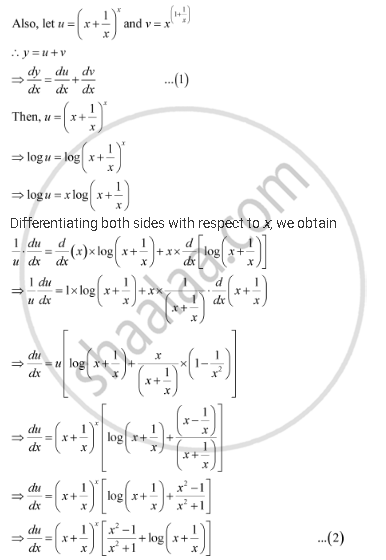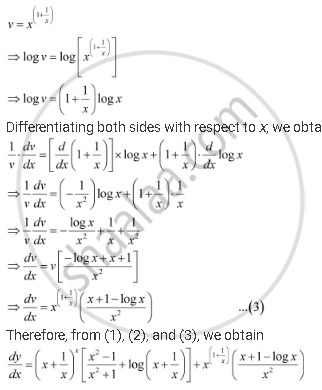Share

# Differentiate the Function with Respect To X. (X + 1/X)^X + X^((1+1/X)) - CBSE (Commerce) Class 12 - Mathematics

#### Question

Differentiate the function with respect to x.

(x + 1/x)^x + x^((1+1/x))

#### Solution

Ley y = (x + 1/x)^x + x^((1+1/x))Is there an error in this question or solution?

#### APPEARS IN

NCERT Solution for Mathematics Textbook for Class 12 (2018 to Current)
Chapter 5: Continuity and Differentiability
Q: 6 | Page no. 178

#### Video TutorialsVIEW ALL 

Solution Differentiate the Function with Respect To X. (X + 1/X)^X + X^((1+1/X)) Concept: Logarithmic Differentiation.
S